﻿ Study on the Engineering Foundation and Structural Scheme of a Foreign-related ProjectPublications are Open
Access in this journal
Article Versions
Export Article
• Normal Style
• MLA Style
• APA Style
• Chicago Style
Case Study
Open Access Peer-reviewed

### Study on the Engineering Foundation and Structural Scheme of a Foreign-related Project

Dong Xiaoli , Zhang Yuhui, Li Rui, Du Jiaming
American Journal of Civil Engineering and Architecture. 2018, 6(5), 216-222. DOI: 10.12691/ajcea-6-5-7
Received August 26, 2018; Revised October 15, 2018; Accepted November 06, 2018

### Abstract

The buildings of the Confucius Institute and the Institute of Media and Art in Mozambique adopt a frame structure. Due to the building function and the architect's personalized modeling requirements, the project has a large planar opening area and a large span structure. We have completed the engineering structural design of the jump column and the foundation design of the geological conditions under which the self-weight collapsible soil. In addition, we also compared and analyzed the reinforcement of the calculation column of the model column method and the modified expansion coefficient method, checked the failure strength and stable ultimate bearing capacity, and also concluded and summarized the results for the design of foreign aid projects. Midas GTS software was used for foundation settlement analysis of self - weight collapsible sand.

### 1. Project Overview

The Confucius Institute and Media Arts College project is located in Mozambique in Maputo, Mozambique mundra (Mozambique's largest public universities) within the university campus, for the two college to build a teaching building, the building area of about 5200m2, four layers on the ground, building height of 14.8 m. See the Figure 1 architectural rendering.

• Figure 1. Architectural rendering

The building is triangular in shape, with 103.2m in width and 66m in depth. The building structure has the following characteristics: (1) The floor area of the building is large, and the area of the mouth of the building has exceeded the 30% requirement stipulated in the building seismic design code (GB 50011-2010) 1, which is determined as a plane irregular structure. (2) There are 14 columns with diameters of 600mm to support the eaves and corridors at the entrance of the teaching building. The horizontal no floor beam is connected to each other, forming an overpass column with a height of 14.8 meters. (3) The project geological condition is relatively complicated, there is self-weight collapsible sand which is evaluated for III class by exploration units.

### 2. Main Structure Analysis

2.1. Design Parameter Settings

The building’s main function is university teaching building, according to the C class architectural design, the seismic fortification intensity is 6 degrees, II site, design earthquake are grouped into the first group, site features period of 0.35s, field roughness category A, basic wind pressure 0.90kN/m2. C30 concrete and steel bar are all made of three-grade steel which strength design value is 360N/mm2.

2.2. Structural Model Calculation

The calculation and analysis of the main structure of this project is conducted by adopting PKPM SATWE software. The span of the main frame beams is 5.5m and 8.8m respectively, and the section sizes are 400x600 and 500x600 respectively. In order to make the displacement angle of the main structure smaller, the larger section size of the frame beam can meet the requirement of maximum displacement ratio of the structure in the case of plane irregularity. As the main structure is irregular on the plane, the SATWE parameters should be selected without using the rigid floor assumption for overall index analysis, so as to ensure the accuracy of the calculation of the actual stiffness of the frame beam and frame column at the entrance. The length coefficient of the external corridor jump column is modified by using the calculation method of SATWE column length (the reference standard of "column length coefficient" is the standard layer column height,that is, the standard layer column "column length coefficient" is 1.0). The length coefficient of the jump column is 14.8 × 1.25/3.6=5.14.

### 3. Slender Column Design

There are 14 columns with diameter of 600mm and height of 14.8 meters at the entrance of the teaching building. According to the code for design of concrete structure (GB 50010-2010) 2 is as follows: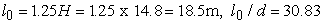(l0 is the length of the column, and d is the diameter of the section)

In engineering, reinforced concrete frame columns are divided into three categories according to the size of l0/d: short column, long column (middle long column) and slender column; l0/d ≤8 is short colum, 8< l0/d≤ 30 is long column; l0/d> 30 is slender column. According to the nature of failure, it can be divided into strength failure and instability failure.

• Figure 2. N-M relationship

Short column in the case of destruction, the lateral displacement of the column close to 0, no buckling, in N - M change stress path in a straight line diagram as shown in Figure 2 (C point) for the strength damage; In the case of destruction, the medium long column slenderness effect can no longer be ignored, although there is a lateral displacement happens, still eventually due to the material reached ultimate strength and failure, but in flexure component produced by the pressure axial second-order effect, can the medium long column under axial pressure than the other conditions the same short column decreases as shown in Figure 2 (A point); Slender column in load under the condition of N - M smaller and medium long column are similar, but when the load reaches a certain critical value, column under axial pressure can increase, the column reached its maximum bearing capacity, as shown in Figure 2 (B point), so the load unchanged, column will continue to increase and into the dynamic deformation and post buckling failure.

The calculation formula of eccentric compression reinforcement is only given in reference 2, without indicating the applicable scope of the formula, or suggesting the risk of instability failure of slender columns. It is necessary to check the strength failure of slender columns and the ultimate bearing capacity of stable failure. The old code for the design of concrete structures (GB 50010-2002) explains the design idea of slender column. When l/h > 30 occurs, due to the reduced strain value of the control section, the steel bar and concrete cannot reach their respective strength design values, which are similar to elastic instability when slender columns fail. The calculation is conducted by formula (7.3.10-1) from GB50010-2002, and the error is large. Model pillars or other reliable methods are recommended for calculation. We will be in accordance with the column "model law" and the literature 3 correction coefficient of expansion method to calculate the slender column reinforcement engineering, and based on the literature 4 calculate formula (4) to check slender column the ultimate bearing capacity of eccentric compression strength failure and stable value, and combining the latest research results of slender column as well as the performance in the main structure of slender column component are summarized.

3.1. Calculation of Reinforcement by "model column" Method

The "model column" method is an approximate algorithm for deflection calculation of slender columns of reinforced concrete, which is proposed by Aas Jakobsen and his son in Norway.

• Figure 3. "model column"

The "model column" method is a cantilever column with a certain curvature (sinusoidal curve) distribution at the bottom of a root solid bearing one-way bending moment (see Figure 3). The curvature distribution during the instability failure makes the column lateral deflection satisfied.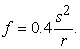(1)

In the formula: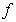is the lateral deflection of the top of the column, starting from the tangent line at the bottom of the column;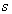is the column length, equal to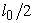,(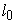the calculated length of slender column);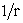is the curvature of the column base section.

• Figure 4. Long column decomposition into two "model columns"

In the actual structure, the columns are decomposed into several "model columns" (eccentrically clamped bars at both ends) according to the constraint conditions of support. As shown in Figure 4, the slender column is decomposed into two model columns, and the maximum displacement of the cross section at the ultimate bearing capacity is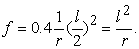(2)

Curvature formula of column base section of section height refers to formula (3) in reference to literature 7: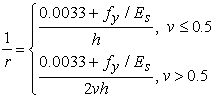(3)

In the formula: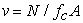is the axial pressure ratio; in the literature 6, it is verified that the effective height of section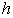is in the curvature formula through theoretical calculation and practical engineering measurement. This paper refers to the practice in the literature 6.

Under the action of the bending moment of the top of the column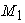, the displacement of the top of the columnand the pressure of the top of the column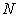,the total bending moment of the column bottom section is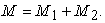(4)

In the formula:is the first order bending moment,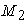is the additional second order bending momentAs shown in Figure 5, the reinforcement calculation results of the column under the control of internal force of the first layer of the project are calculated.

Because of the symmetry of the circular section, the bending moment in both directions can only be considered within the same quadrant. The bending moment takes the absolute value and is substituted into the above formula to obtain: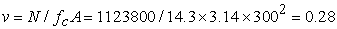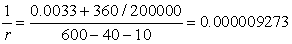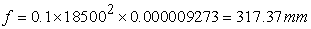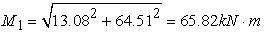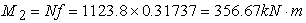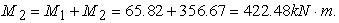The ratio of eccentricity to effective radius of the circular section is calculated: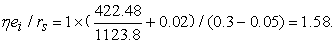Based on the calculation results, we refer to the literature 7 to find The approximate ratio of the central angle of the area of the concrete column section to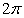is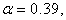parameters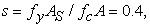then the area of all longitudinal reinforcement in the section is: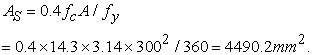The results of reinforcement are modified according to literature 7: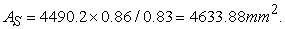• Figure 5. Reinforcement calculation results of STAWE column controlling internal force
3.2. Modified Expansion Coefficient Method for Reinforcement Calculation

The modified expansion coefficient method is a special research group to carry out the experiment of eccentric force members for 194 rectangular cross sections of both ends of hinge branches and 33 circular and ring cross sections. The member is 9 < l/h < 50. And the computer is used to analyze the whole force of the column and use the simplified formula to correct the expansion coefficient.

The ratio of the eccentricity to the effective radius of the circular section, that is, in reference 7, where the coefficient of eccentricity increase is taken as formula (5) in reference 3: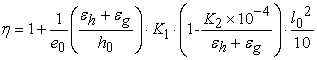(5)

Where,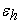- Concrete compression strain,=0.0033;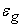- The strain of steel bar with tension edge is described in section 6.2.3 according to article 2 in literature, take=0.01;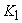- Considering the influence of load eccentricity on the curvature of column section, when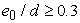,=1，when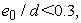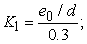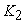- Considering the influence coefficient of the curvature of the column section with long and thin ratio, when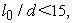=0, when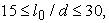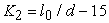, when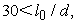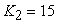;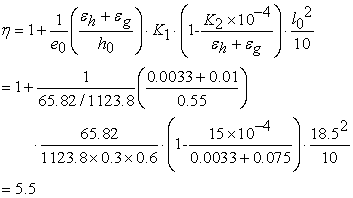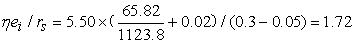According to the calculation results in the literature 7 shows the approximate ratio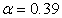of the central angle of the area of the concrete column section to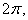parameter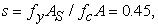and the area of all longitudinal reinforcement of the section: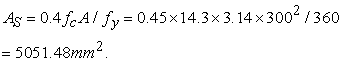The results of reinforcement are modified according to literature 7,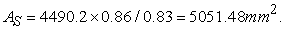Comparing the "model column" method with the modified expansion coefficient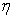method, there is no significant difference in the calculation results of the two slender columns. The arrangement of reinforcement should be based on the equal spacing of longitudinal reinforcement and the spacing should not be greater than 200mm, 12 steel bars with diameter of 25mm can be configured. The actual amount of reinforcement is 5890.8mm2.

3.3. Strength Failure and Stability Failure Limit Bearing Capacity Calculation

The strength failure and stability failure ultimate bearing capacity of the reinforced concrete bars with eccentric compression on slender columns are checked in accordance with the formula (6) in reference to literature 4. The analysis results can be referred to literature 8. The formula is: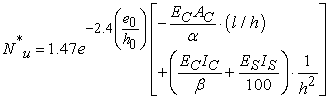(6)

Where: --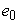axial pressure on section center eccentricity (mm);-- height of section (mm);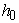-- effective height of section (mm);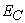-- the elastic modulus of concrete shall be taken in accordance with the code for design of concrete structures (N/mm2);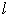-- length of column (mm);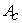-- gross area of sectional concrete (mm2);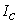-- moment of inertia of concrete gross section (mm4);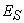-- elastic modulus of longitudinal reinforcement (N/mm2);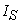-- the moment of inertia of the steel bar section (mm4) is calculated by multiplying the area of each side of the steel bar by the square of its distance from the geometric center of the section.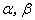-- constants, which are obtained according to Table 1.

By the calculation,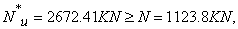then the column will not experience strength failure or stability failure, but it is not difficult to find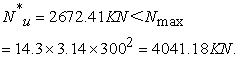The maximum bearing capacity of slender column is still decreased when the axial pressure is low.

3.4. Summary of Slender Column Research Results

Combined with this paper and literature 10, 11, the following conclusions can be obtained:

(1) In the design of slender columns, even if the axial pressure ratio of slender columns is small, the results of column reinforcement calculated by the structural design specification method are much less than those calculated by the "model column" method and the modified expansion coefficient method (in the case of this paper, the area of the former reinforcement is only 51.8% of that of the latter two methods). In the case of complex force, two methods can be used to calculate, and the results of calculation are relatively large.It is necessary to calculate the strength failure of slender column and the ultimate bearing capacity of stable failure.

(2) In the case of the same cross-section reinforcement or axial pressure ratio, the higher the column height, the higher the yield displacement Angle and ultimate displacement angle, the better the deformation performance of the cross-section column.

(3) When the same cross section and reinforcement, with the increase of earthquake effect, ordinary column first appeared at the end of the yield tensile damage occurs, the layer column tensile damage degree is always lagging behind the ordinary post, ordinary column to achieve ultimate displacement angle damage occurs, the interlayer displacement angle of the layer column is always less than the interlayer displacement angle of common column, the trend is not affected by the structure of beam-column stiffness difference. The seismic performance of the overpass column is better than that of the ordinary column.

### 4. Foundation Design of Collapsible Sand

The distribution of soil and the characteristic value of soil bearing capacity are shown in Table 2. According to the geotechnical engineering survey report of this project, the degree of subsidence of the soil in layer 2 and 3 is classified as medium. The total collapsibility of the soil is greater than 30cm, which should be considered as the gravity collapsibility site, and the scollapsibility level of the soil is defined as grade III.

4.1. Design of Foundation

The grouting pile is used in the foundation scheme, and the 6th layer of fine sand and silty sand is used as the bearing layer of pile. Factors to be considered in this scheme: (1) The local structure span is large, and the concentrated load on the bearing part of the large span is high, resulting in large difference in the distribution of building load. The problem of building differential settlement coordination on the same base is prominent. (2) The proposed site is a self-weight collapsible site, the second and third layers of sand have self-weight collapsibility, low bearing capacity and soil layer distribution depth of about 10m. (3) According to the technical specification for building pile foundation (JGJ 94-2008) 11 3.4.2, the design concept of collapsible site is the principle of "passing through".

4.2. Design Details of Pile Foundation

(1) According to the engineering anti-floating fortification level, after the completion of the project won't rise to the level 2 water in the sand, but if improper protection during construction, there might be 2, 3 layer saturated situation, with reference to the literature 11, with the 2, 3 layers of collaspsibility sand as a saturated state, pile side resistance should be taken into account by the negative, it is disadvantage for pile foundation. Therefore, it is suggested that when the characteristic value of pile lateral friction resistance is evaluated, the values of fine sand and silt in layers 2 and 3 are as follows: qsik= -10kpa.

(2) According to the data collected during the professional investigation and detailed investigation, no post-grouting technology of bored cast-in-place piles has been used in the project in Maputo, and the local engineering geological background information is scarce, without any engineering experience. At the same time, the engineering for the foreign aid project, carrying out survey work lack the required tools and test equipment is not fully evaluation work of collapsibility of soil, so the related strata after grouting pile foundation design phase coefficient βsi, the resistance of lateral resistance enhancement coefficient of βp can reference 11, and taking a conservative values, lateral resistance enhancement coefficient of βsi take 1.5, end resistance enhancement coefficient of βp take 2.0 , and requirement of the characteristic value of vertical bearing capacity of the single pile static load test, and the pile number at least accounted for 5% of the total number of piles, the final determination of pile static load test of single pile bearing capacity can satisfy the design requirements.

(3) Check calculation analysis of pile foundation settlement

By using the PKPM STAWE foundation design function to import geological survey data, calculate and analyze the foundation settlement contour line (see Figure 6), and find out the pile position with the maximum settlement difference (see Figure 7). The settlement is 3.45mm and 0.28mm respectively, and the settlement difference is 3.17mm=0.0006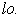As the PKPM cannot fully and effectively simulate special soil, Midas GTS software is used to analyze and calculate the foundation settlement. The maximum settlement difference pile foundation model and calculation results are shown in Figure 8 and Figure 9. The settlement is 6.85mm and 1.64mm, respectively. The settlement difference is 5.21mm=0.001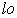. Settlement amount and settlement difference and pile distance meet the standard requirements.

• Figure 6. STAWE subsidence contour analysis
• Figure 7. STAWE maximum differential settlement pile location
• Figure 8. Maximum differential settlement of MIDAS GTS pile model
• Figure 9. Maximum differential settlement of MIDAS GTS pile location settlement

### 5. Conclusion

Through the project research, the following three conclusions can be drawn, which can provide reference and have important practical value for the engineering practice in the slender over-storey column design and the foundation design under self-weight collapsibility site.

(1) This project is a plane irregular structure, so reasonable structural joints should be adopted to divide the building into regular structures. At the same time, the larger cross-section size of the seismic components is selected to make the displacement angle of the main structure smaller, so as to meet the requirement of the maximum displacement ratio of the structure in the case of plane irregularity. In addition, the structural measures such as increasing the thickness of the slab, setting dark beams at the edge of the hole, and setting inclined steel bars in the corner of the opening of the floor are adopted to improve the weakening of the stiffness of the floor and the irregularity of the plane. (2) For the over-storey column with a diameter of 600mm and a height of 14.8 meters at the entrance of the teaching building, the reinforcement calculation of the jump column was carried out by using the "model column" method and the modified expansion coefficient method, and the research results of the jump column were summarized to check the strength failure and stability failure limit bearing capacity, which ensured the safety of the jump column in the structure. (3) The project is located at the collapsible site under gravity weight, and special design is carried out for the foundation engineering. In view of the particularity of soil engineering, the parameters such as the characteristic value of pile lateral friction resistance qsik, the coefficient of lateral resistance βsi, the coefficient of end resistance βp , etc., were adjusted during foundation design.The settlement of the foundation is analyzed and calculated with a variety of software, and the safety of the design of the foundation scheme is verified.

### References

  GB 50011-2010 Building Seismic Design Code of the Ministry of Construction of the People's Republic of China. Beijing: China Building Industry Press, 2010. In article  GB 50010-2010 Concrete Structure Design Specification of the Ministry of Construction of the People's Republic of China. Beijing: China Building Industry Press, 2010. In article  Wei Wei, Du Jing, Bai Shaoliang. Strength Failure and Stability Failure Ultimate Bearing Capacity Analysis of Slender and Eccentric Reinforced Concrete Bars. 2006. 23 (4).114-119. In article  Calculation of Eccentrically Compressed Long Column of Reinforced Concrete, Thematic Research Group of Eccentrically Compressed Components. In article  Wang Yiqun, Sun Fuping. Calculation of Reinforcing Bars for Bidirectional Eccentric Compression Reinforced Concrete Cylindrical Slender Columns. Architectural Structure, 2008, 38 (10): 99-101. In article  Zhang Li, Li Ziqing, Hu Zhaotong, et al. Numerical Method for Solving the Curve of Cylindrical Reinforced Concrete Bridge Pier. Journal of Xi'An Highway and Communications University, 2001, 21 (3); 45-119. In article  Lu Zhitao, Yang Xiaofeng. Simple and Practical Calculation of Normal Section Force of Reinforced Concrete Bars with Circular and Circular Sections. Architectural Structure, 1996, 26 (5): 16-24. In article  Liu Yi. Discussion of Several Problems in Second-Order Effect and Stability of Reinforced Concrete. Chongqing, Chongqing University of Architecture, 2000. In article  Zheng Yimin, Wang Dong. Discussion on Seismic Performance of Jump Columns. Structural Engineer, 2012, 28 (2): 89-94. In article  Zheng Yimin, Duan Xingyu. Study on Seismic Performance of Jump Column Based on ABAQUS Damage Plasticity Model. Structural Engineer, 2014, 30 (1): 93-100. In article  JGJ 94-2008 Building Pile Technical Specification, Ministry of Construction, People's Republic of China. Beijing: China Building Industry Press, 2008. In article

Published with license by Science and Education Publishing, Copyright © 2018 Dong Xiaoli, Zhang Yuhui, Li Rui and Du JiamingThis work is licensed under a Creative Commons Attribution 4.0 International License. To view a copy of this license, visit http://creativecommons.org/licenses/by/4.0/